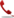+91-9818369374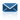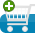Ask an Expert for Free Submit Your Assignment/Home work1 Live Tutoring Free Trial / 30 Min Sample Assignment / Home Work Make Payment Course Offered Resources
Quick Contact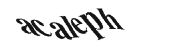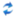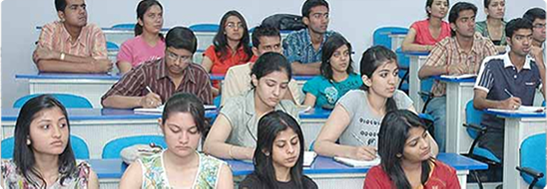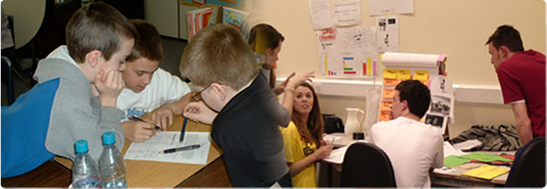# Question

A triangle has sides of length 4, 5, 7 units. Find, to the nearest tenth of a degree, the size of the largest angle.

(Total 4 marks)

2.       O is the centre of the circle which has a radius of 5.4 cm.The area of the shaded sector OAB is 21.6 cm2. Find the length of the minor arc AB.

(Total 4 marks)

3.       The circle shown has centre O and radius 6.is the vector,is the vectorandis the vector.(a)     Verify that A, B and C lie on the circle.

(3)

(b)     Find the vector.

(2)

(c)     Using an appropriate scalar product, or otherwise, find the cosine of angle.

(3)

(d)     Find the area of triangle ABC, giving your answer in the form a, where a Î.

(4)

(Total 12 marks)

4.       Solve the equation 3 sin2 x = cos2 x, for 0° £ x £ 180°.

(Total 4 marks)

5.       The diagrams show a circular sector of radius 10 cm and angle θ radians which is formed into a cone of slant height 10 cm. The vertical height h of the cone is equal to the radius r of its base. Find the angle θ radians.